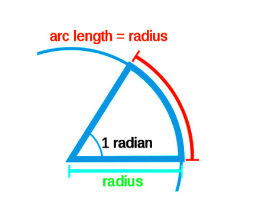A radian is defined as the angle of an arc in a circle that is created by enclosing the radius of the circle around its circumference. We represent the angle between two lines through radians and degrees. The total angle of a circle equals 360 or we can call it as 2 radians. With the help of radians to degrees we can convert the angles that are represented in radians into degrees.Radians to Degrees Formula is given as,

$\large Degrees=\frac{Radians\times 180}{\pi}$

### Solved Examples

Question: Convert 1.46 radians to degrees?
Solution:
The formula to convert radians to degrees is,
Degrees = $\frac{Radians*180}{π}$

Degrees = $\frac{1.46*180}{π}$

Degrees = 83.69

#### 1 Comment

1. Daniel Omwansu Asigo

Good I like it